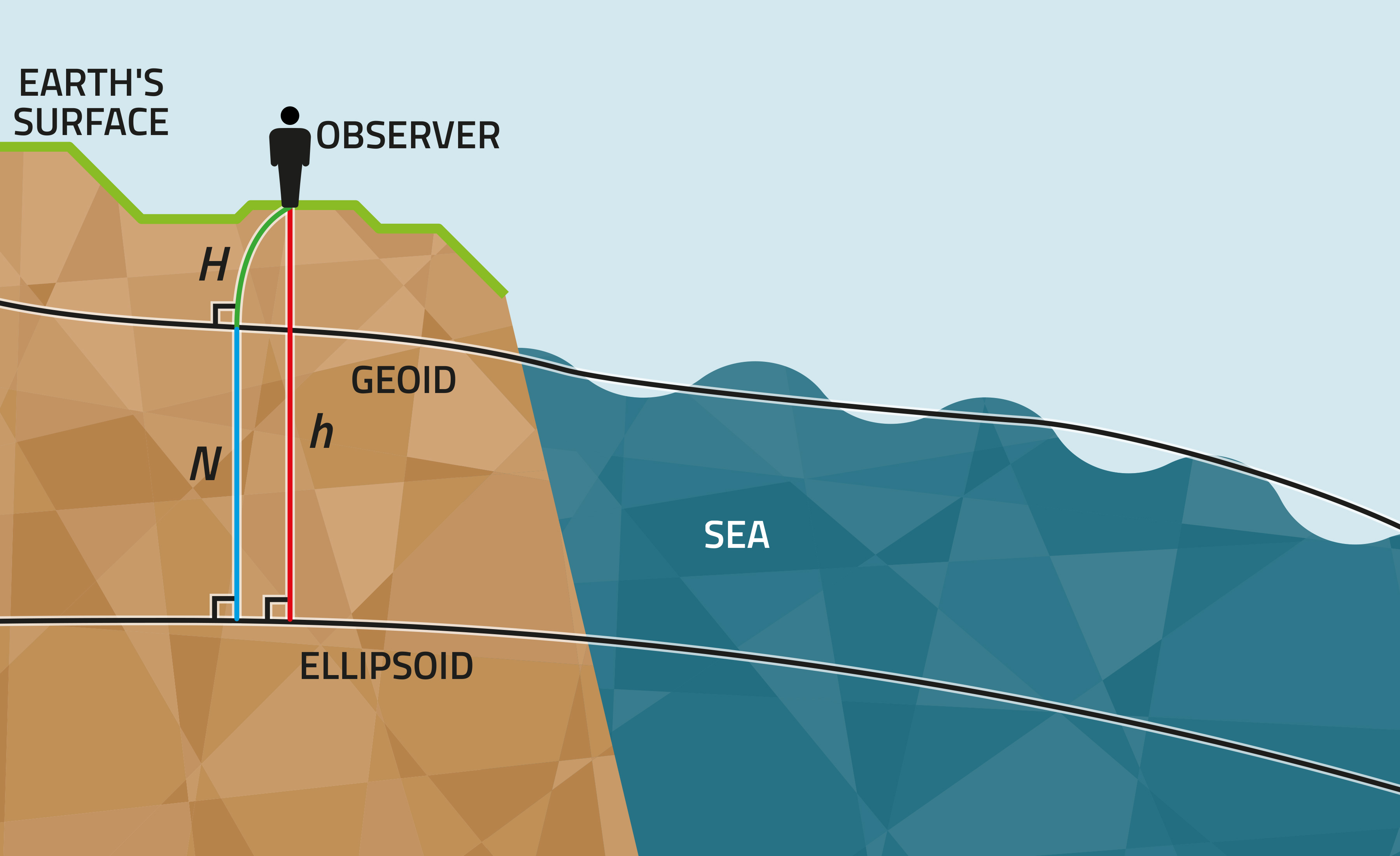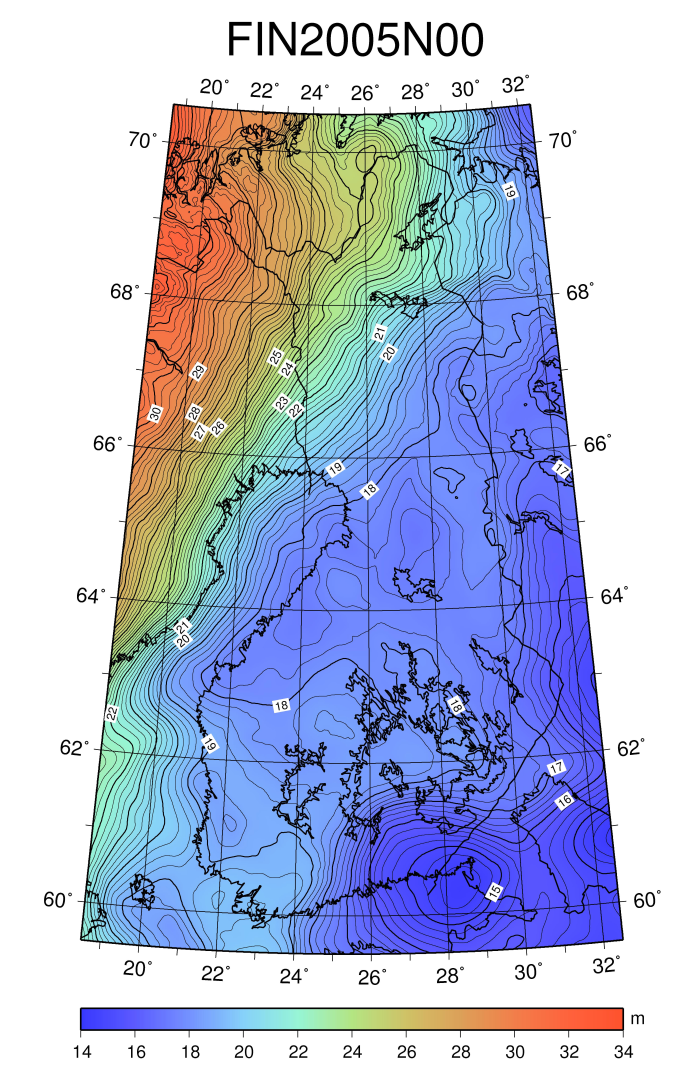# Geoid

The geoid is the shape that describes the Earth and the shape the oceans would take at rest. The geoid surface connects the oceans and continues through the continents. In reality, the mean sea level deviates from the geoid due to variations in the ocean's temperature, salinity, and air pressure as well as prevailing winds and ocean currents.Figure 1. The relationship between geoid height (N), ellipsoidal height (h), and levelled height (H) is h = H + N.

It is hard to mathematically determine the geoid's exact shape and position in 3D space because the geoid is a physical surface defined by the spatial changes in the Earth's masses and densities. The mass of the Earth is unevenly distributed, resulting in a wavy geoid surface. The geoid surface is further from the centre of the Earth in some places than in others. These variations are very small in comparison with the Earth's radius.

The spatial changes in the geoid surface (the geoid undulations) are studied internationally using satellites. The global gravity field is mapped by Earth-orbiting satellites. This gravity data, obtained by satellites, is refined with gravity measurements made on the Earth's surface. For example, the GOCE gravity satellite of the European Space Agency (ESA) studies the Earth's gravitational field and geoid.Figure 2. This map shows the geoid heights with respect to the Earth’s ellipsoid.

The geoid is usually given as heights with respect to a reference ellipsoid, and in that case, we talk about a geoid model. Geoid models are calculated using gravity observations. National geoid models are obtained by fitting a local geoid model to national reference systems. The geoid model for Finland is FIN2005N00. Heights obtained with satellite positioning, EUREF-FIN ellipsoidal heights, can be transformed to levelled N2000 heights using the model. The FIN2005N00 geoid model can be found at our website (in Finnish).

# Mirjam Bilker-Koivula

D.Sc. (Tech.)
Research Group Manager